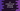# C# program to get the substring of a string## C# program to get the substring of a string:

In C#, we can use Substring method of string to get one substring. This method has two different variants. Following are these methods:

``````String.Substring(start)
String.Substring(start, length)``````

We can use both of these methods to get the substring of a string in C#.

### String.Substring(start):

This method takes only one parameter, the start index for the sub-string. It returns a substring starting from the start index to the end of the given string.

It will throw one ArgumentOutOfRangeException if the start index provided is less than 0 or if it is greater than the length of the string.

### Example of String.Substring(start):

Let’s take a look at the below example:

``````using System;

namespace Demo
{
public class Program
{
public static void Main(string[] args)
{
string given_str = "Hello World !!";
Console.WriteLine(given_str.Substring(3));
Console.WriteLine(given_str.Substring(0));
Console.WriteLine(given_str.Substring(6));
}
}
}``````

If you run this, it will give the below output:

``````lo World !!
Hello World !!
World !!``````

Here,

• The first statement returns the substring from index 3 to the end of the string.
• The second statement returns the substring from index 0 to end, i.e. the complete string.
• The third statement returns the substring from index 6 to end.

### String.SubString(start, length):

This methood takes two parameters. The first one is the start index or the index in the string to start the sub-string and the second one is the length of the sub-string. For invalid start and length, it throws ArgumentOutOfRangeException.

Let’s take a look at the example below:

``````using System;

namespace Demo
{
public class Program
{
public static void Main(string[] args)
{
string given_str = "Hello World !!";
Console.WriteLine(given_str.Substring(0, 4));
Console.WriteLine(given_str.Substring(0, 0));
Console.WriteLine(given_str.Substring(0, 1));
}
}
}``````

This program will print the below output:

``````Hell

H``````

Here,

• The first statement is getting a sub-string from index 0 of size 4
• The second statement printed an empty string as the length is 0.
• The third statement printed H as the length is 1 and we are starting from the start of the string.